# [Example 2] Suspended Material Transport in a Simple Bed Flume

In this section, we perform the following computations using a simple curved flume with straight inlet out let parts. The Cross section of the flume is composed with a compound channel in which both the low water channel and the flood plane with moveable bed. Then flood plane is located only left side of the low water channel. The experiment was carried out by CTI Engineering Co. Ltd. on behalf of Civil Engineering Research Institute of Cold Region . A movie taken from a drone during the experiment is shown in Figure 51, and the experimental condition and plane and cross sectional view pictures are shown in Figure 52.

The computational exercises in this section is conducted as the following procedure.

• Flow and bed deformation by Nays2DH until the bed reaches an equilibrium state

• Quasi 3-dimensional flow field by Nays2d+

• Tracer tracking by UTT. Check the effect of turbulent diffusivity by changing parameter

## Calculation of Flow and bed deformation by Nasy2DH

### Select a Solver

From the iRIC startup screen, click [Create New Project], and select [Nays2dH iRIC3x 1.0 64bit] in the Figure 53.

A window titled as「Untitled- iRIC 3.x.xxxx [Nays2DH iRIC3X 1.0 64bit]」appears.

### Grid Creation

Select from the main menu [Grid]->[Select Algorithm]. Then a window appears as Figure 55, select [2d arc grid generator (Compound Channel)] and click [OK].

In the [Groups] of the [Grid Creation] window, set parameters of, [Channel shape], [Cross section], [Additional Channel] and [Roughness and fixed/moveable bed] as, Figure 56 , Figure 57 , Figure 58 , and Figure 59 , respectively.

When you finished all the settings of the grid creating condition, click [Create Grid] in the above grid creating condition windows, e.g. Figure 59. After clicking [Create Grid] button, you will be asked [Do you want to map?], then answer [Yes], and the computational grid is created. ( Figure 60 )

Put check marks in [Grid], [Cell Attributes] and [Fixed or Moveable bed] in the object browser, Figure 61 appears with the fixed bed part in red and the moveable bed part shown in blue.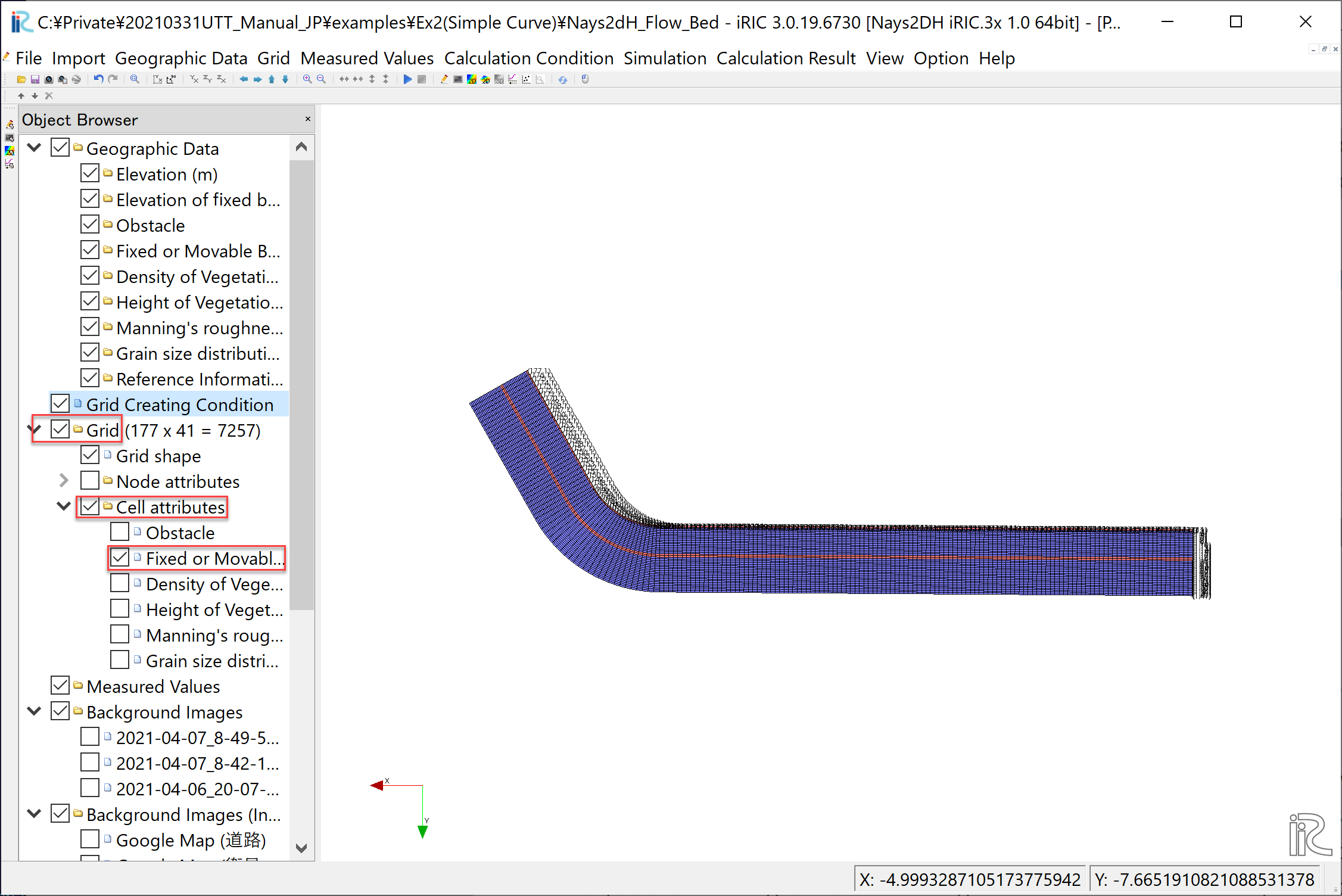Figure 61 : Grid Shape with Fixed and Moveable bed Colored

The red part of the fixed bed along the boundary between the low water channel and the flood plane is assumed to be a revetment, in this grid creating tool, however, since the revetment in the actual experiment is only the bend part plus short length of upstream and downstream. So, as shown in Figure 62, focus [Fixed or Moveable bed], and right-click on a straight section of the revetment part (in this case, the red section upstream of grid number 87) and change the attribute to [Moveable bed], and press [OK].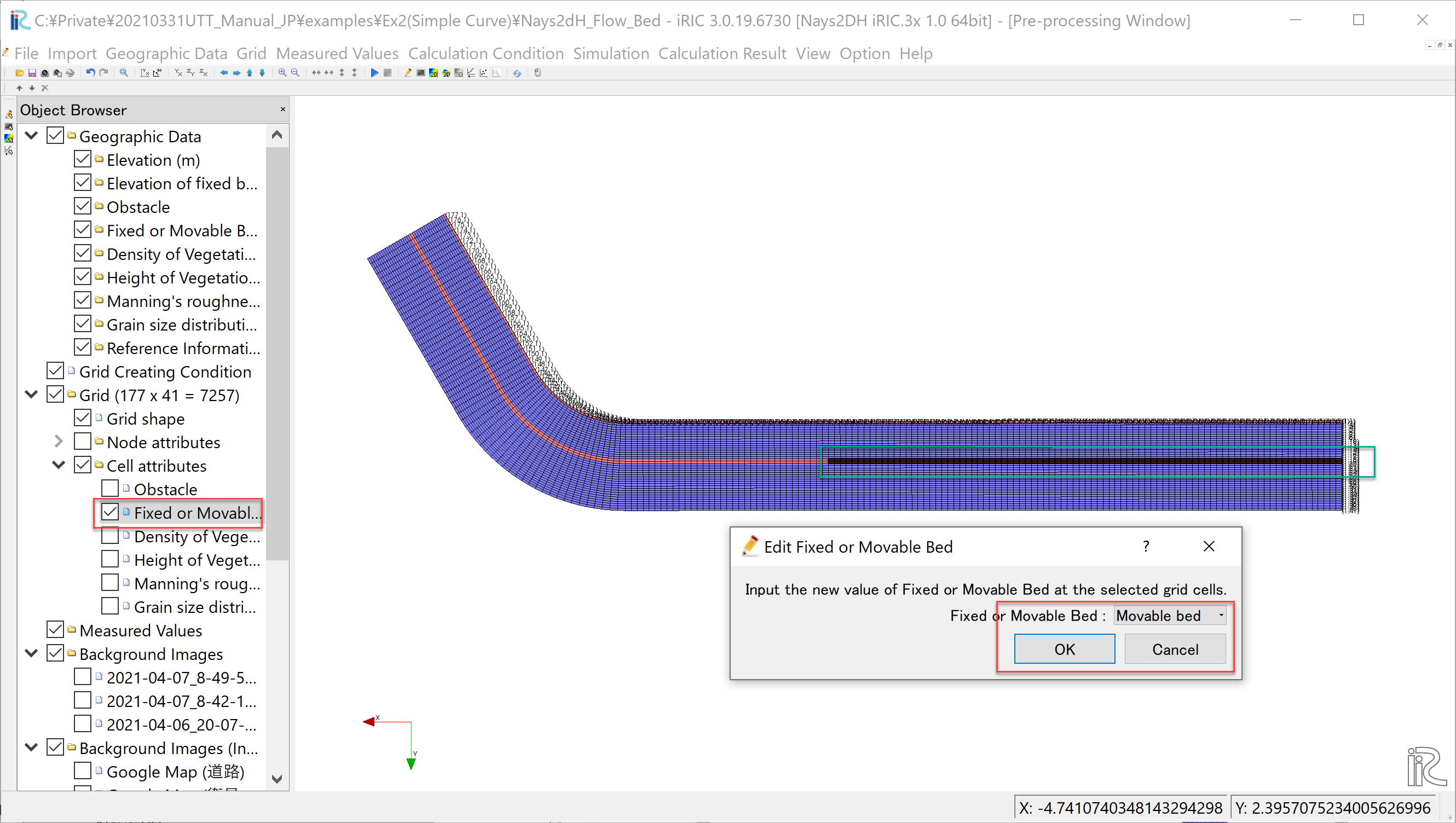Figure 62 : Change attribute from fixed bed to moveable bed

Since the downstream end is the fixed bed, set the attribute of the downstream end cells into [Fixed Bed], by expanding and rotating, as demonstrated in Figure 63.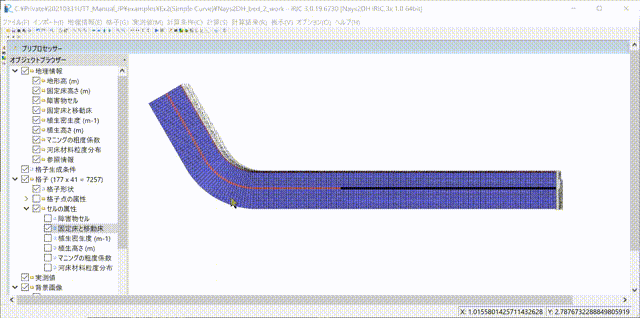Figure 63 : Change downstream end cell attribute to fixed bed

### Setting Computational Condition

Show the [Calculation Condition] window by selecting [Calculation Condition]->[Setting], and in the [Group] of [Solver Type], [Boundary Condition], [Time] and [Bed Material] , set the parameters, as Figure 64 , Figure 65 , Figure 66 , and Figure 67, respectively.

In addition, in the [Boundary Condition] setting of Figure 65, press  of [Time series of discharge at upstream end ……], and set [Time] and [Discharge] hydrograph data in the [Time series of discharge at upstream end ……] window as Figure 68, and press [OK].

When you finished the settings of all the computational condition parameters, press [Save and Close] in the [Calculation Condition] window.

### Run Nays2DH

Before executing the Nays2DH, select [File]->[Save as Project] and save the project. Here we save the project as a name of [Nays2DH_flow_bed] (Figure 69)

From the main menu, select [Simulation]->[Run], then a window asking [Do you want to save?] appears as Figure 70. Then press [Yes], save as a project, and the computation starts running as Figure 71.

When the computation finished, save the results by selecting [Calculation Result]->[Save], from the main menu.

### Display the Calculation Results

Open a [Post Processing Window] by selecting [Calculation Result]->[Open new 2D Post-Processing Window] as Figure 72.

In the object browser of the [Post Processing Window], put check marks in [iRICZone], [Scalar(node)] and [ElevationChange(m)], right click [ElevationChange(m)] to show [Property] and press it, open [Scalar Settings], and set parameters as Figure 73.

In the object browser, put check marks in [Arrow] and [Velocity(m)], right click [Arrow], show [Property] and press it, open [Arrow Setting Window] as Figure 74, and set parameters as marked with red squares in the Figure 74.

Put the [Time Scale Bar] back to zero, select [Animation]->[Srart/Stop] to start animation as Figure 75.

As shown in Figure 76, it is shown that the bed elevation change reached an equilibrium.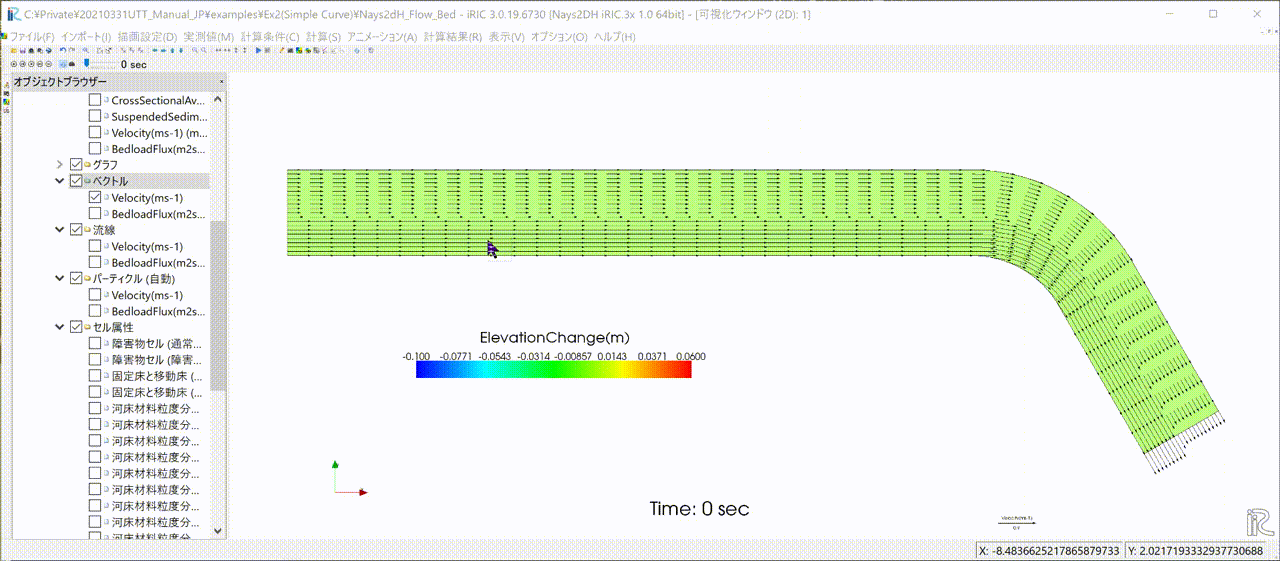Figure 76 : Animation of velocity vectors and bed elevation changes

### Export the Computational Results

In order to use the calculated bed elevation as an boundary conditions for the quasi-3D flow calculation by Nays2d+ in the next section, we export the calculated results to a text file. As shown in Figure 77, select [File]->[Export]->[Calculation Result].

When the [Export Calculation Result] setting window (Figure 78) is appeared, choose [Format] as [Topography Files(*.tpo)].

The output folder can be any name, and uncheck the checkbox at [All timesteps], and set [Start] and [End] as 7,200. Then click [OK] to complete the export of the calculation Results Figure 79.

The exported calculation results are stored in the specified folder. As shown in Figure 80, many files contain different values as water depth, velocity, sediment transport rate, riverbed elevations, and so on, however, since only the riverbed elevation is used for the flow calculations in the next section, all files except [Result_1_Elevation(m).tpo] can be deleted.

## Quasi-3D Flow Calculation by Nays2d+

### Selecting a Solver

From the iRIC startup screen, click [Create New Project], and select [Nays2d+] in the Figure 81, and press [OK].

### Importing Computational Grid, Channel Bed Elevation and Mapping

#### Importing Grid

From the main menu, select [Import]->[Grid], and choose [Case1.cgn] in the folder of [Nays2DH_floe_bed] which was created in the previous section. While importing, a warning as Figure 82 is coming out, press [Yes], and complete importing grid (Figure 83).

#### Import Bed Elevation

From the main menu, select [Import]->[Geographic Data]->[Elevation](Figure 84).

In the import file selection window, Figure 85, assign the file [Results_1_Elevation(m).tpo], which was exported from Nays2dH calculated results in the previous section.

Figure 86 appears, but if there is no particular need to thin out the data, you can leave it as it is, and press [OK] to complete the import the [Bed Elevation] (Figure 87).

#### Execute Mapping

The imported bed elevation data is mapped onto the imported computational grid. Select [Grid]->[Attribute Mapping]->[Execute] as Figure 88.

As Figure 89, you will be asked which [Geographic Data] to be mapped. Put check mark in the box of [Elevation(m)], and press [OK].

When the mapping is completed, press [OK] as Figure 90.

### Setting Calculation Condition for Nays2d＋

In the window of [Calculation Condition] which appears when you select [Calculation Condition]->[Setting], set parameters in the [Groups] of [Discharge and downstream water surface elevation], [Time and bed erosion parameters], [Boundary Condition], [Other computational parameters] and [3D Velocity Profile] as, Figure 91, Figure 92, Figure 93, Figure 94, and Figure 95, respectively.

In addition, while in the settings of the [Discharge and downstream water surface elevation], Figure 91, press  and set discharge data in in the [Time series of discharge and downstream stare] setting window as Figure 96.

When you finish setting all the calculation condition, press [Save and Close] in the [Calculation Condition] window.

### Execute Nays2d+

We will skip the explanation of how to executing Nays2d+ because it is exactly same as other solvers. However, it is recommended that you save the project before running the calculation. In this case, we save the file to a project named [Nays2d+Flow].

The results are saved in a CGNS file named [Case1.cgn], which will be used for the tracer tracking computation of UTT as input data. Be sure to save the result using [Calculation Result]->[Save] even when the calculation is finished. (Figure 98).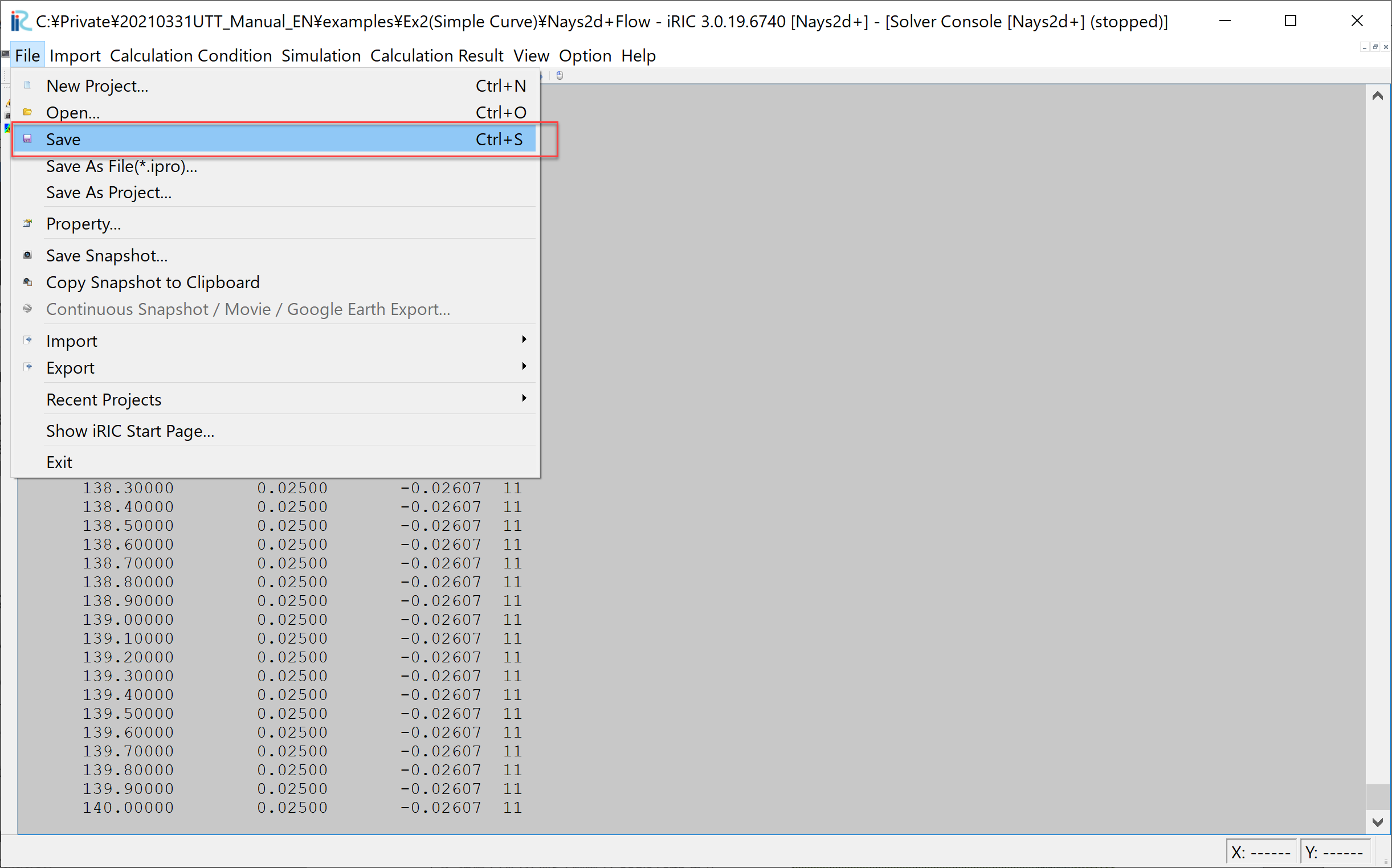Figure 98 : Save the Results of the Computation (Don’t Forget!)

## Tracer Tracking by UTT

### Select a Solver

From the iRIC startup screen, select [New Project], and in the solver selection screen appears. Select “UTT” and click “OK” (Figure 99).

### Import Grid

Right click [Grid(No Data)] and select [Import] as Figure 100.

From the [Select Import File] window as Figure 101, choose [Case1.cgn] in the folder [Nays2d+Flow] which is produced by the [Nays2d+] calculation in the previous section.

Press [Yes] button when warning message is coming out as Figure 102, and the grid import is completed as Figure 103.

### Tracer Tracking Simulation by UTT

#### Setting Simulation Condition

From the main menu bar, when you select [Calculation condition]->[Setting]. [Calculation Condition] window appears, and in this window, set parameters in the [Groups] of [Basic Settings], [Normal Tracers Supplying Condition] and [Diffusion Condition], as Figure 104, Figure 105, and Figure 106, respectively. In this section, we first perform tracer tracking without considering the effect of sub-grid turbulence.

In addition.Figure 100 The [CGNS file to read the flow calculation results] in the [CGNS file to read the flow calculation results] is the same as the one in the previous section [Flow calculation with Nays2d+]. Select [Case1.cgn] in the [Nays2d+Flow] project folder where you saved the results of ( Figure 103)

In addition, the [Flow information input file] in Figure 104, is the same file with the [Case1.cgn] which was produced by the flow simulation of [Nays2d+] in the previous section (Figure 107).

#### Run UTT

From the main menu, select [Simulation]]->[Run], then you will be asked to save project as usual, save project as recommended. ( Figure 108).

In the Figure 109, either [Save as file (*.ipro)] or [Save as Project] will do, but in this example, the file is saved as [UTT1.ipro].

When the computation starts, Figure 110 appears, and when the computation finishes, Figure 111 appears. Press [OK] to finish computation.

#### Showing the Results of UTT

From the main menu, select [Calculation Result]->[Open new 2D Post Processing Window], and the calculation results are shown (Figure 112)

Since the orientation of the Figure 112 is the opposite to the experimental image shown at the beginning of this chapter Figure 51, press the 90° rotation mark twice to rotate 180° (Figure 113).

Since the [Time] display is so small that it’s hard to see, select [Time]->[Properties] in the object browser (Figure 114), display [Time Setting] and set the font size appropriately large (Figure 115).

As shown in Figure 116, put time bar back to 0, and from the main menu, select [Animation]->[Start/Stop], then the animation starts( Figure 117).

There is almost no diffusion and the tracers are just flowing straightly.

#### Comparison of the Turbulent Diffusivity

Select [Calculation Condition]->[Setting] and open [Calculation Condition] window. As shown in Figure 118, set in the [Group]->[Diffusion Condition], [Diffusivity Correction]->[Yes] and set the value [A=1] of the [Diffusivity Parameter]

In the same manner, if we do the simulation with [A=5], [A=10] and [A=50], the results becomes as Figure 120, Figure 121 and Figure 122.

When we compared with the experimental results of the Figure 51, it seem that the case with A=10, Figure 121, is the closest to the experiment.

#### Cloning of the Tracers

In the main menu, select [Calculation Condition]->[Setting] to show [Calculation Condition]. In the [Calculation Condition] window, select [Tracer Cloning and Amalgamation], set parameters as Figure 123. Select [Diffusion Condition] and set [A=1] and press[Save and Close] as Figure 124. Then execute the UTT solver by choosing [Simulation]->[Run], and show the results (Figure 125).

The spread range of the tracers in Figure 51 is close to the diffusion range of the green dye in the experimental movie. The number of tracers appears to be enormous, but if you put check marks in [Particles]->[Scalars]->[Generations] in the object browser, generations of the tracers are displayed as Figure 126.

When this is animated, it becomes as Figure 127.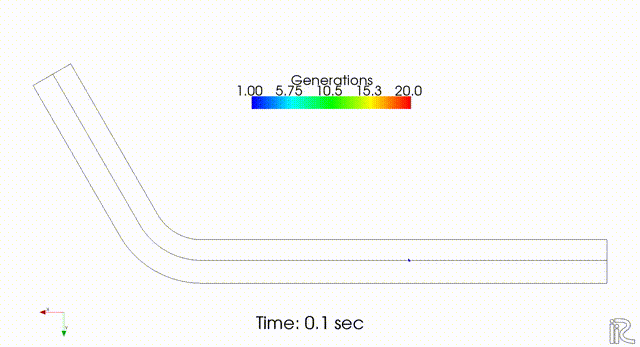Figure 127 : Tracers Clone Animation(Maximum 20 Generations, A=10, Color-coded View)

As described in Overview , the substantial weight in the 10th generation is W=0.00195, and in the 20th generation is W=0.00000195. Therefore, Figure 126, the concentrations of the tracers of green, yellow, red, etc. are logarithmically lower than that of the central blue tracers. To see the real concentration, the substantial concentration in each cell is visualized by the following procedure.

1. Uncheck the check box at [Scalar] in the object browser (Figure 128).

2. Put check mark at [Scalar(Cell Center)] and [Weighted numbers of tracers] in the Object Browser (Figure 129).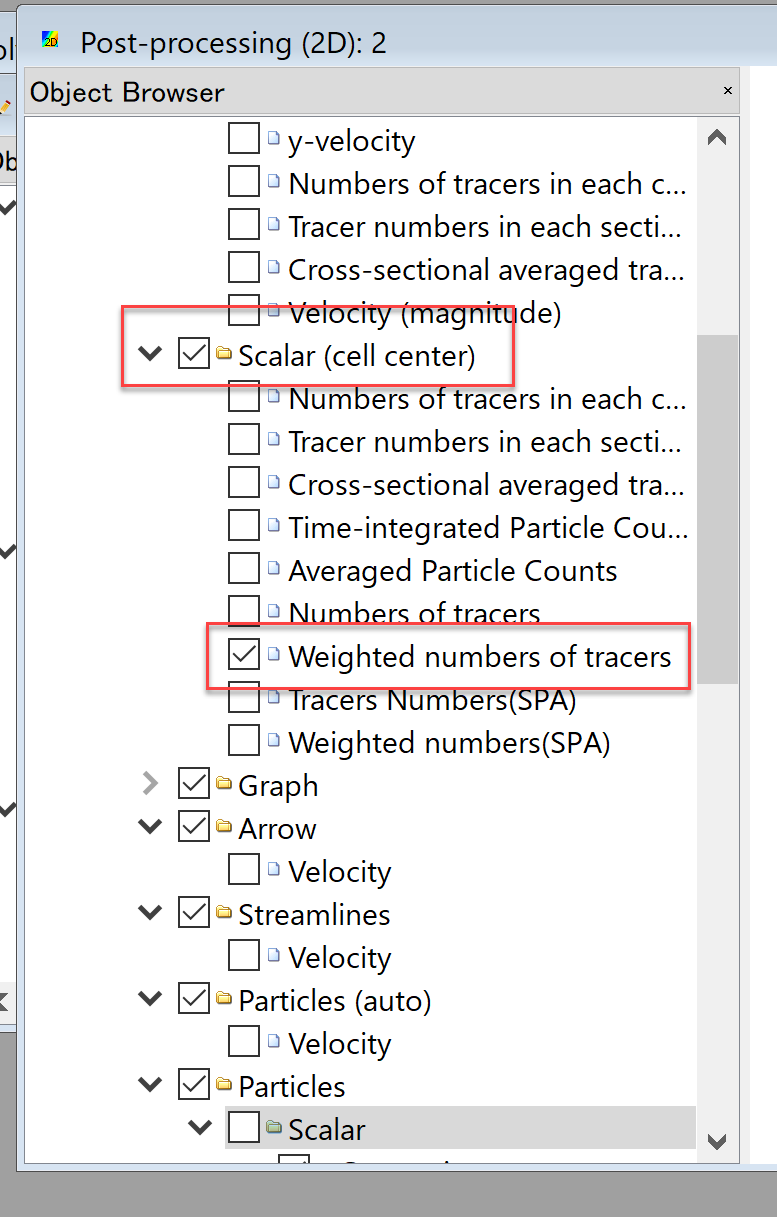Figure 129 : Put check mark at [Weighted numbers of tracers]
1. Right click [Weighted numbers of tracers] and press [Property]

4. In the [Scalar Setting] window, uncheck mart at [Auto], set [Max=0.1] and [Min61e-08], remove the check mark at [Fill Lower Area], set [Color map] as [Manual], and press [Setting].

5. In the [Custom Color Map] window, set [Type] [3 Colors], [Maximum Value] as [Dark Green], [Medium Value] as [Light Green] and [1e-06], [Minimum Value] as [White] and press [OK].

1. When the window got back to the [Scalar Settings], press [OK] to finish.

In the [Post processing (2D)] window, Figure 134, put time bar back to zero, select [Animation]->[Start/Stop], and start animation as Figure 135.

The diffusion situation is similar to that of the green dye in the experimental movie of Figure 51.

### Flow Visualization using Tracer Cloning

Flow visualization using tracer cloning is shown in this section. In the main menu, click [Calculation Condition], and set parameters in the [Group] of [Normal Tracers Supplying Condition] and [Tracer Cloning and Amalgamation] as Figure 136 and Figure 137, respectively, and press [Save and CLose].

Figure 136

Then after running the UTT solver. in the [Object Browser], remove check mark from [Weighted numbers of tracers], put check marks in boxes at [Particles], [Scalar] and remove the check mark form the [Generation].

From the main menu, select [Animation]->[Srat/Stop], and the animation with evenly distributed tracers in the whole channel is visualized.

### Swimming Fish Simulation

Set the following parameters in the [Computation of Fish Motion] in the [Calculation Condition] window menu followed by selecting [Calculation Condition]->[Setting] in the main menu.

After setting these parameters, run the solver by [Simulation]->[Run]. Once close the existing [2D Post-processing 2D window], open a new [2D Post-processing 2D window], put check mark on [Polygon]->[Fish]->[Type] as Figure 142, and select [Animation]->[Start/Stop]. Then Figure 143 is played.

## Driftwood Tracking by NaysDW2 and Visualization

In this section, driftwood tracking simulation by NaysDW2 (Nays Driftwood 3D) is shown.

### Select a Solver

From the iRIC startup screen, click [Create New Project], and select [NaysDw2(Simple 2D Driftwood Tracker)] as shown in Figure 144, and press [OK].

### Import Computational Grid

As shown in Figure 145, from the [Object Browser], right click [Grid(No data)], and press [Import]

When the file selection window appears, select [Case1.cgn] in the [Nays2d+Flow] folder in which the computational results of the [Nays2d+] stored. (Figure 146)

Neglect the waring message as Figure 102, press [Yes], and the grid importing is completed (Figure 148).

### Setting Condition

From the main menu, select [Calculation Condition]->[Setting],and set the calculation condition as follows.

In the [Calculation Condition] window, press file selection bar as Figure 149.

In the [Select File] window, Figure 150, select [Case1.cgn] which contains the calculation results of the [Nays2d+] in the previous section.

Set other parameters in [Basic Setting] as Figure 151.

Set parameters in [Driftwood Feeding Condition] as Figure 152.

Set [Flow and driftwood condition] parameters as Figure 153, and press [Save and Close].

### Run Driftwood Simulation

From the main menu, select [Simulation]->[Run] as Figure 154.

When you are asked [Do you want to save?] as Figure 155, press [Yes] and save the project.

As Figure 156, when you are asked [How to save the project], in this example, select [Save as project], and press [OK].

As Figure 157, choose an empty folder to save project, and press [Select Folder].

When the calculation starts, Figure 158 is displayed, and Figure 159 is appear when the calculation ends. Then click [OK] to finish calculation.

### Visualization of driftwood motion

From the main menu, select [Calculation Result]->[Open New 2D Post-processing Window] as Figure 160.

In the [Object Browser] of Figure 161, put check marks in the boxes at [iRICZone], [Scalar(node)] and [res_Velocity(magnitude)], right click [res_Velocity(magnitude)] and choose [Property].